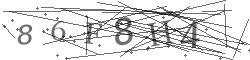# Current report Return >

## 2016

Number Date Subject
57/2016 2016-09-28 Notification on change of shares

The Management Board of Boryszew S.A. (the company) announces that the company received the

notification from Mr. Roman Krzysztof Karkosik informing that on 23 September, 2016 he purchased in

transactions on the Warsaw Stock Exchange 114,551 units of shares of Boryszew S.A. (settlement of

the transaction was 27 September, 2016) and on 26 September, 2016 he purchased in block trades

250,000 units of shares of Boryszew S.A. (settlement of the transaction was 27 September, 2016).

The above mentioned transactions have resulted in change of indirectly share in the total number of

votes at GM of Boryszew S.A. by 1 %.

Before the transaction – on 22 September, 2016 Mr. Roman Krzysztof Karkosik owned directly

126,686,953 units of shares of Boryszew S.A., which constituted 52.786% of equity and indirectly

through subsidiaries 19,200,000 units of shares of Boryszew S.A., which constituted 8.00% of equity.

The possessed shares have given directly 126,675,056 votes at the GM of Boryszew S.A., which are

equal to 52.781% of the total number of votes at the GM and indirectly through subsidiaries

19,200,000 votes at the GM of Boryszew S.A., which are equal to 8.00% of the total number of votes

at the GM, including:

 Alchemia S.A.: 3,200,000 units of shares, which constituted 1.333% of equity; 3,200,000 votes

at the GM, which are equal to 1.333% of the total number of votes at the GM,

 Boryszew S.A.: 7,830,000 units of shares, which constituted 3.262% of .equity; 7,830,000

votes at the GM, which are equal to 3.262% of the total number of votes at the GM,

 Impexmetal S.A.: 6,000,000 units of shares, which constituted 2.50% of equity; 6,000,000

votes at the GM, which are equal to 2.50% of the total number of votes at the GM,

 SPV Boryszew 3 Sp. z o.o: 2,165,000 units of shares, which constituted 0.902% of equity;

2,165,000 votes at the GM, which are equal to 0.902% of the total number of votes at the GM,

 Polski Cynk Sp. z o.o.: 5,000 units of shares, which constituted 0.002% of equity; 5,000 votes

at the GM, which are equal to 0.002% of the total number of votes at the GM.

On 27 September, 2016 Mr. Roman Krzysztof Karkosik owned directly 127,079,460 units of shares of

Boryszew S.A., which constituted 52.9497% of equity and indirectly through subsidiaries 19,200,000

units of shares of Boryszew S.A., which constituted 8.00% of equity.

The possessed shares have given directly 127,001,504 votes at the GM of Boryszew S.A., which are

equal to 52.917% of the total number of votes at the GM and indirectly through subsidiaries

19,200,000 votes at the GM of Boryszew S.A., which are equal to 8.00% of the total number of votes

at the GM, including:

 Alchemia S.A.: 3,200,000 units of shares, which constituted 1.333% of equity; 3,200,000 votes

at the GM, which are equal to 1.333% of the total number of votes at the GM,

 Boryszew S.A.: 7,830,000 units of shares, which constituted 3.262% of .equity; 7,830,000

votes at the GM, which are equal to 3.262% of the total number of votes at the GM,

 Impexmetal S.A.: 6,000,000 units of shares, which constituted 2.50% of equity; 6,000,000

votes at the GM, which are equal to 2.50% of the total number of votes at the GM,

 SPV Boryszew 3 Sp. z o.o: 2,165,000 units of shares, which constituted 0.902% of equity;

2,165,000 votes at the GM, which are equal to 0.902% of the total number of votes at the GM,

 Polski Cynk Sp. z o.o.: 5,000 units of shares, which constituted 0.002% of equity; 5,000 votes

at the GM, which are equal to 0.002% of the total number of votes at the GM.

Signature:

Piotr Szeliga – President of the Management Board

Translation from the original Polish version.

In the event of differences resulting from the translation, reference should be made to the official

Polish version.

Up

If you have Any questions, please fill out the form. We will reply as soon as we can.Refresh